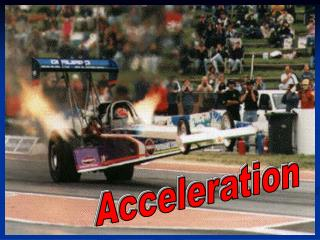DownloadDownload PresentationAcceleration

# Acceleration

Télécharger la présentation## Acceleration

- - - - - - - - - - - - - - - - - - - - - - - - - - - E N D - - - - - - - - - - - - - - - - - - - - - - - - - - -
##### Presentation Transcript

1. Acceleration

2. Bell Ringer Sports announcers will occasionally say that a person is accelerating if he/she is moving fast. Why is this not an accurate description of what acceleration is?

3. Objectives Define acceleration in words and with an equation Identify positive and negative acceleration by analyzing a graph Calculate acceleration with the use of an equation and graph Define and apply the force of gravity

4. Change in Velocity • Each time you take a step you are _________________ the _____________ of your body. • You are probably most familiar with the velocity changes of a moving bus or car. • The rate at which _________ (__________ or __________) changes occur is called _______________________.

5. Acceleration= ________ ______ ________ ______ Change in velocity = final – starting velocity velocity Acceleration= __________________________

6. A car traveling at 60 mph accelerates to 90 mph in 3 seconds. What is the car’s acceleration? Velocity(final) - Velocity(original) = Acceleration time = = =

7. A car traveling at 60 mph slams on the breaks to avoid hitting a deer. The car comes to a safe stop 6 seconds after applying the breaks. What is the car’s acceleration? Velocity(final) - Velocity(original) = Acceleration time = = =

8. - A _________________ acceleration produces a __________ line or linear slope (_______________). - The ________ of a non-linear velocity-time graph (rise/run) will predict an objects instantaneous ____________________. a = / Calculate the instantaneous acceleration of the graph: a = v/t a = a =

9. Free fall • The constant ___________________ of an object moving only under the force of _______________ is "g". • The acceleration caused by ____________ is _____ m/s2 • If there was no _________, all objects would fall at the same ____________ • Doesn’t depend on ____________ • After 1 second falling at 10 m/s • After 2 seconds ________ m/s • 3 seconds _________ m/s

10. Galileo • 1600’s • Studied how things fell • Didn’t have a good clock • Rolled balls down an _______________ plane • Found that the ___________ increased as it rolled __________ the ramp

11. t = 0 t = 1 second t = 2 seconds t = 3 seconds Galileo Acceleration= ____________________________

12. Galileo • Same things happen when things fall • Didn’t drop things from Tower of Pisa

13. Falling • ______ resistance will increase as it falls ______________ • An ________________ force on the object • Eventually __________ will ______________ with _______ resistance • Reaches _____________ velocity - highest speed reached by a falling object.

14. Terminal velocity • Force of gravity is constant • air resistance ___________ as you speed up • until the force is _________ • _____________ forces, no ________________________ • ______________ velocity _______________ velocity

15. _______________ Velocity and _____________ Acceleration

16. __________________ Velocity and ______________ Acceleration

17. _________________ Velocity and ______________ Acceleration